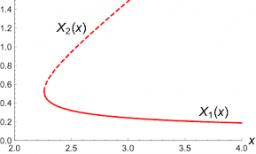# Equations 5070

Solve in RxRxR a system of 3 linear equations with three unknowns:

1/2 x + 3/4 y = 6z
2x-z = 10 1/2
2z + x = 2y + 7
note : / is a fraction line

x =  5.4545
y =  -0.3636
z =  0.4091

### Step-by-step explanation:

1/2 x+3/4 y=6z
2x-z=10 + 1/2
2z+x=2y+7

1/2·x+3/4·y=6·z
2·x-z=10 + 1/2
2·z+x=2·y+7

2x+3y-24z = 0
4x-2z = 21
x-2y+2z = 7

Pivot: Row 1 ↔ Row 2
4x-2z = 21
2x+3y-24z = 0
x-2y+2z = 7

Row 2 - 2/4 · Row 1 → Row 2
4x-2z = 21
3y-23z = -10.5
x-2y+2z = 7

Row 3 - 1/4 · Row 1 → Row 3
4x-2z = 21
3y-23z = -10.5
-2y+2.5z = 1.75

Row 3 - -2/3 · Row 2 → Row 3
4x-2z = 21
3y-23z = -10.5
-12.833z = -5.25

z = -5.25/-12.83333333 = 0.40909091
y = -10.5+23z/3 = -10.5+23 · 0.40909091/3 = -0.36363636
x = 21+2z/4 = 21+2 · 0.40909091/4 = 5.45454545

x = 60/11 ≈ 5.454545
y = -4/11 ≈ -0.363636
z = 9/22 ≈ 0.409091

Our linear equations calculator calculates it.Did you find an error or inaccuracy? Feel free to write us. Thank you!

Tips for related online calculators
Do you have a system of equations and looking for calculator system of linear equations?